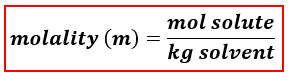# Problem: A solution was prepared by dissolving 39.0 g of KCl in 225 mL of water.  Calculate the molality of KCl in the solution.

###### FREE Expert Solution

We’re being asked to calculate for the molality of the solution prepared by dissolving 39.0 g of KCl in 225 mL of water

When calculating for molality, we use the following equation:We will calculate the molality of the solution using the following steps:

Step 1: Calculate the moles of solute.
Step 2: Calculate the mass of the solvent (in kg).
Step 3: Calculate the molality of the solution.

KCl → dissolved in water → Solute

Water (H2O) → where KCl is dissolved → Solvent

Step 1: Calculate the moles of KCl.###### Problem Details

A solution was prepared by dissolving 39.0 g of KCl in 225 mL of water.  Calculate the molality of KCl in the solution.